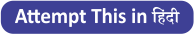Latest Banking jobs   »   Reasoning Ability Quiz For IBPS RRB...

# Reasoning Ability Quiz For IBPS RRB PO, Clerk Prelims 2021- 3rd June

Directions (1-5): In this question, relationship between different elements is shown in the statements. The statements are followed by conclusions. Study the conclusions based on the given statement and select the appropriate answer.

Q1. Statements: A≥B=Q≤P<J≤Y; Z≥A>X; A<M
Conclusions:
I.B<Y
II.X≥J
(a) Only conclusion I is true.
(b) Neither conclusion I nor II is true.
(c) Only conclusion II is true.
(d) Both conclusions I and conclusion II are true.
(e) Either conclusion I or II is true.

Q2. Statements: M>A≥B=Q; Z≥A>X; Q≤P<J≤Y
Conclusions:
I.Z=Q
II.Z>Q
(a) Only conclusion I is true.
(b) Neither conclusion I nor II is true.
(c) Only conclusion II is true.
(d) Both conclusion I and conclusion II are true.
(e) Either conclusion I or II is true.

Q3. Statements: T<R; X<G<R=A≤S
Conclusions:
I.G<S
II.S>T
(a) Only conclusion I is true.
(b) Neither conclusion I nor II is true.
(c) Only conclusion II is true.
(d) Both conclusions I and conclusion II are true.
(e) Either conclusion I or II is true.

Q4. Statements: M<K≤I>N; D≥P; I≥C; P=U<M
Conclusions:
I.M<C
II.N>U
(a) Only conclusion I is true.
(b) Neither conclusion I nor II is true.
(c) Only conclusion II is true.
(d) Both conclusions I and conclusion II are true.
(e) Either conclusion I or II is true.

Q5. Statements: P=U<M<K≤I>N; D≥P;I≥C
Conclusions:
I.D≥K
II.I>P
(a) Only conclusion I is true.
(b) Neither conclusion I nor II is true.
(c) Only conclusion II is true.
(d) Both conclusions I and conclusion II are true.
(e) Either conclusion I or II is true.

Q6. Which of the following symbols should replace the question mark in the given expression to make A ≥ D definitely true?
A= G ≥ B ? F ? M = D
(a) >, >
(b) =, >
(c) ≤, ≤
(d) ≤, <
(e) None of these

Q7. Which of the following symbols should replace the question mark(?) in the given expression in order to make the expressions A < B definitely true and D ≤ A definitely true?
B ? E = A ? F ≥ N = D
(a) >, ≤
(b) =, >
(c) <, >
(d) >, =
(e) ≤, <

Q8. Which of the following symbols should replace the question mark in the given expression to make N ≥ D definitely true?
A= G ≥ B, N ? F ? M = D, B > N
(a) >, ≥
(b) =, >
(c) ≤, ≤
(d) ≤, <
(e) None of these

Q9. Which of the following symbols should replace the question mark(?) in the given expression in order to make the expressions M < E definitely false and B ≤ A definitely true?
M ? B ? E = A
(a) >, ≤
(b) =, >
(c) <, >
(d) =, =
(e) ≤, <

Q10. Which of the following symbols should replace the question mark in the given expression to make G ≥ F and M <B definitely true?
A? G ? B ? F ? M
(a) >, >,>, ≤
(b) =, >,>, ≤
(c) ≤, ≤,>, ≤
(d) ≤, <,>, ≤
(e) None of these

Directions (11-15): In these questions, relationship between different elements is shown in the statements. The statements are followed by conclusions. Study the conclusions based on the given statements and select the appropriate answer. Give answer-
(a) If only conclusion II is true
(b) If only conclusion I is true
(c) If both conclusions I and II are true.
(d) If either conclusion I or II is true
(e) If neither conclusion I nor II is true.

Q11. Statements: A>G<J, I≤D, J<O=K>D.
Conclusions:
I. G>D
II. O>I

Q12. Statements: P≥W≤Z, W>K, Q>O<Z
Conclusions:
I. W>Q
II. Z>K

Q13. Statements: B≥L<J>O, E>Y<L
Conclusions:
I. B>Y
II. J>Y

Q14. Statements: T>O<A<Q≥M>N<V
Conclusions:
I. T>N
II. A>M

Q15. Statements: B>Q≥R, Z>T≥R>J
Conclusions:
I. B > J
II. Z > Q

Practice More Questions of Reasoning for Competitive Exams:

###### Study Plan for IBPS RRB PO/Clerk Prelims 2021

SolutionsSolution (1-5):
S1.Ans.(a)
S2.Ans.(e)
S3.Ans.(d)
S4.Ans.(b)
S5.Ans.(c)

S6. Ans (e)
S7. Ans (d)
S8. Ans (e)
S9. Ans (a)
S10. Ans (e)

Solutions (11-15):
S11. Ans.(a)
Sol. I. G>D (False) II. O>I (True)

S12. Ans.(a)

Sol. I. W>Q(False) II. Z>K (True)

S13. Ans.(c)

Sol. I. B>Y(True) II. J>Y (True)

S14. Ans.(e)

Sol. I. T>N (False) II. A>M (False)

S15. Ans.(b)

Sol. I. B > J (True) II. Z > Q (False)